Publicité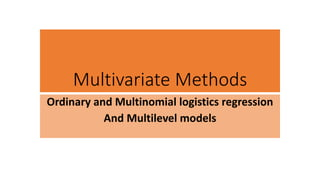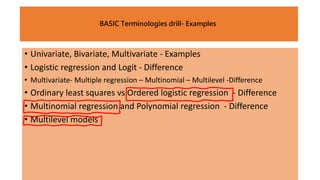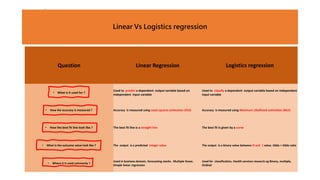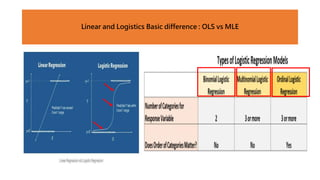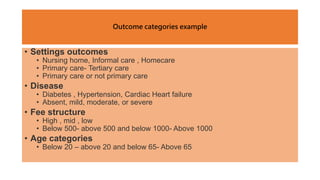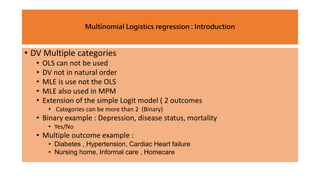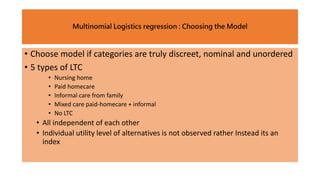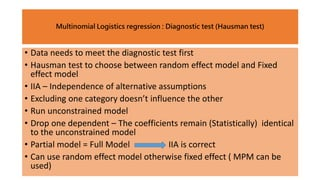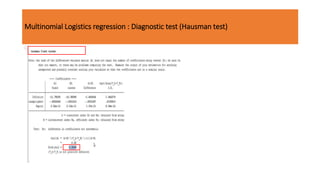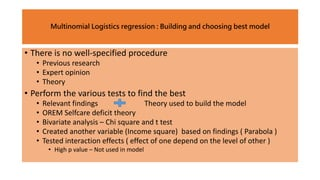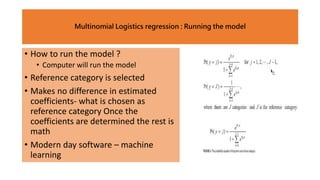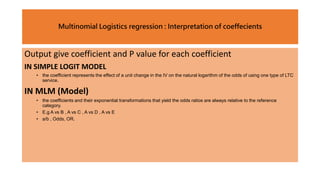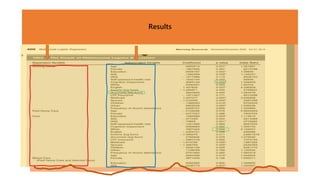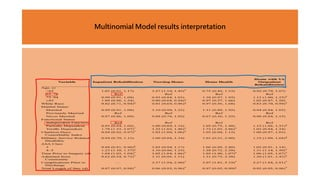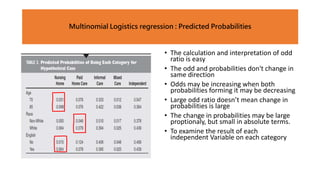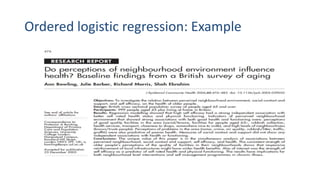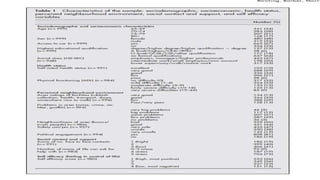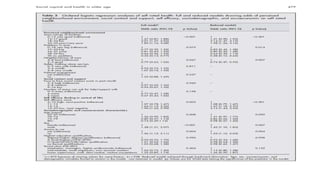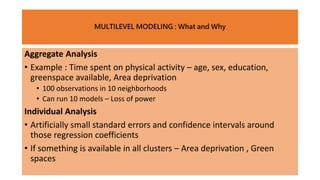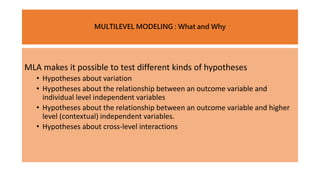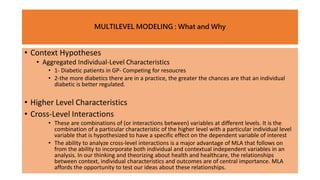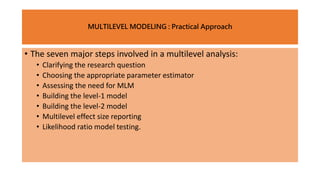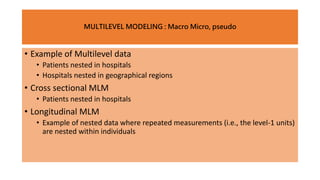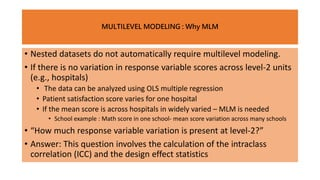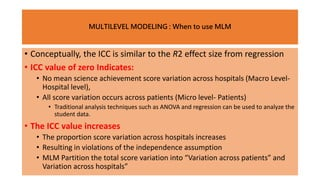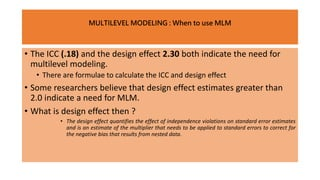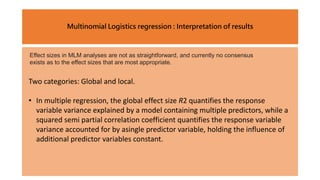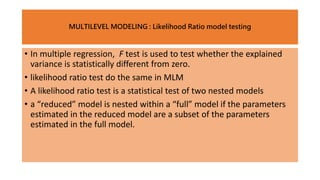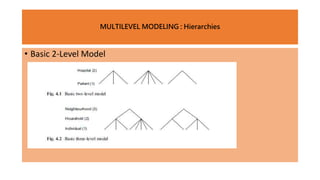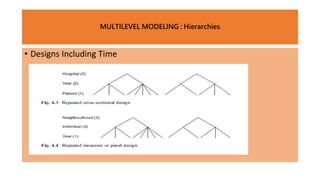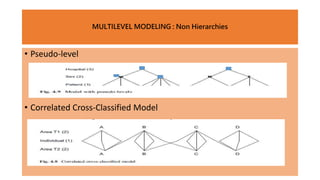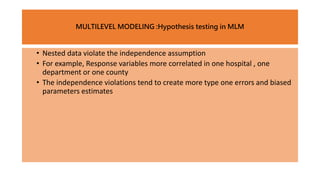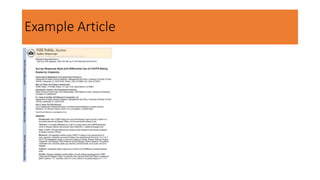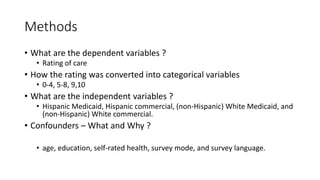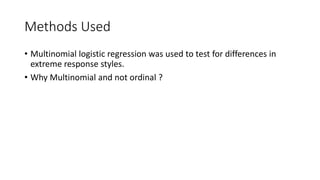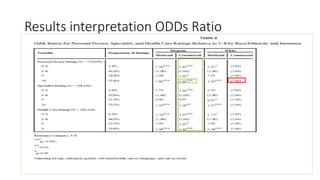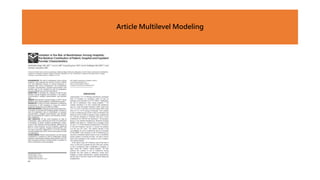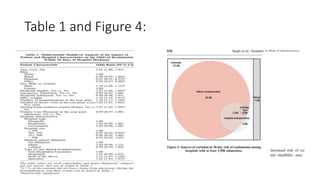1 sur 39
Publicité

### RM MLM PPT March_22nd 2023.pptx

1. Multivariate Methods Ordinary and Multinomial logistics regression And Multilevel models
2. BASIC Terminologies drill- Examples • Univariate, Bivariate, Multivariate - Examples • Logistic regression and Logit - Difference • Multivariate- Multiple regression – Multinomial – Multilevel -Difference • Ordinary least squares vs Ordered logistic regression - Difference • Multinomial regression and Polynomial regression - Difference • Multilevel models BASIC Terminologies drill- Examples
3. Linear Vs Logistics regression Question Linear Regression Logistics regression • What is it used for ? Used to predict a dependent output variable based on independent input variable Used to classify a dependent output variable based on Independent input variable • How the accuracy is measured ? Accuracy is measured using Least squares estimation (OLS) Accuracy is measured using Maximum Likelihood estimation (MLE) • How the best fit line look like ? The best fit line is a straight line The best fit Is given by a curve • What is the outcome value look like ? The output is a predicted integer value The output Is a binary value between O and 1 value. Odds > Odds ratio • Where it is used commonly ? Used in business domain, forecasting stocks . Multiple linear, Simple linear regression Used for classification, Health services research eg Binary, multiple, Ordinal
4. Linear and Logistics Basic difference : OLS vs MLE
5. Outcome categories example • Settings outcomes • Nursing home, Informal care , Homecare • Primary care- Tertiary care • Primary care or not primary care • Disease • Diabetes , Hypertension, Cardiac Heart failure • Absent, mild, moderate, or severe • Fee structure • High , mid , low • Below 500- above 500 and below 1000- Above 1000 • Age categories • Below 20 – above 20 and below 65- Above 65
6. Multinomial Logistics regression : Introduction • DV Multiple categories • OLS can not be used • DV not in natural order • MLE is use not the OLS • MLE also used in MPM • Extension of the simple Logit model ( 2 outcomes • Categories can be more than 2 (Binary) • Binary example : Depression, disease status, mortality • Yes/No • Multiple outcome example : • Diabetes , Hypertension, Cardiac Heart failure • Nursing home, Informal care , Homecare
7. • Choose model if categories are truly discreet, nominal and unordered • 5 types of LTC • Nursing home • Paid homecare • Informal care from family • Mixed care paid-homecare + informal • No LTC • All independent of each other • Individual utility level of alternatives is not observed rather Instead its an index Multinomial Logistics regression : Choosing the Model
8. • Data needs to meet the diagnostic test first • Hausman test to choose between random effect model and Fixed effect model • IIA – Independence of alternative assumptions • Excluding one category doesn’t influence the other • Run unconstrained model • Drop one dependent – The coefficients remain (Statistically) identical to the unconstrained model • Partial model = Full Model IIA is correct • Can use random effect model otherwise fixed effect ( MPM can be used) Multinomial Logistics regression : Diagnostic test (Hausman test)
9. Multinomial Logistics regression : Diagnostic test (Hausman test)
10. • There is no well-specified procedure • Previous research • Expert opinion • Theory • Perform the various tests to find the best • Relevant findings Theory used to build the model • OREM Selfcare deficit theory • Bivariate analysis – Chi square and t test • Created another variable (Income square) based on findings ( Parabola ) • Tested interaction effects ( effect of one depend on the level of other ) • High p value – Not used in model Multinomial Logistics regression : Building and choosing best model
11. • How to run the model ? • Computer will run the model • Reference category is selected • Makes no difference in estimated coefficients- what is chosen as reference category Once the coefficients are determined the rest is math • Modern day software – machine learning Multinomial Logistics regression : Running the model
12. Output give coefficient and P value for each coefficient IN SIMPLE LOGIT MODEL • the coefficient represents the effect of a unit change in the IV on the natural logarithm of the odds of using one type of LTC service. IN MLM (Model) • the coefficients and their exponential transformations that yield the odds ratios are always relative to the reference category. • E.g A vs B , A vs C , A vs D , A vs E • a/b , Odds, OR. Multinomial Logistics regression : Interpretation of coeffecients
13. Results
14. Wald test Wald test is used to compare models on best fit criteria in case of logistic regression. This technique is used to determine 'significant' variables from the set of predictors used in to a variety of models with binary variables or models with continuous variables. Likelihood ratio test The Likelihood-Ratio Test (LRT) is a statistical test used to compare the goodness of fit of two models based on the ratio of their likelihoods. Multinomial Logistics regression : Predicted Probabilities and analysis of results
15. Multinomial Model results interpretation
16. • The calculation and interpretation of odd ratio is easy • The odd and probabilities don't change in same direction • Odds may be increasing when both probabilities forming it may be decreasing • Large odd ratio doesn’t mean change in probabilities is large • The change in probabilities may be large proptionaly, but small in absolute terms. • To examine the result of each independent Variable on each category Multinomial Logistics regression : Predicted Probabilities
17. Ordered logistic regression: Example
18. MULTILEVEL MODELING : What and Why Aggregate Analysis • Example : Time spent on physical activity – age, sex, education, greenspace available, Area deprivation • 100 observations in 10 neighborhoods • Can run 10 models – Loss of power Individual Analysis • Artificially small standard errors and confidence intervals around those regression coefficients • If something is available in all clusters – Area deprivation , Green spaces
19. MULTILEVEL MODELING : What and Why MLA makes it possible to test different kinds of hypotheses • Hypotheses about variation • Hypotheses about the relationship between an outcome variable and individual level independent variables • Hypotheses about the relationship between an outcome variable and higher level (contextual) independent variables. • Hypotheses about cross-level interactions
20. MULTILEVEL MODELING : What and Why • Context Hypotheses • Aggregated Individual-Level Characteristics • 1- Diabetic patients in GP- Competing for resoucres • 2-the more diabetics there are in a practice, the greater the chances are that an individual diabetic is better regulated. • Higher Level Characteristics • Cross-Level Interactions • These are combinations of (or interactions between) variables at different levels. It is the combination of a particular characteristic of the higher level with a particular individual level variable that is hypothesized to have a specific effect on the dependent variable of interest • The ability to analyze cross-level interactions is a major advantage of MLA that follows on from the ability to incorporate both individual and contextual independent variables in an analysis. In our thinking and theorizing about health and healthcare, the relationships between context, individual characteristics and outcomes are of central importance. MLA affords the opportunity to test our ideas about these relationships.
21. MULTILEVEL MODELING : Practical Approach • The seven major steps involved in a multilevel analysis: • Clarifying the research question • Choosing the appropriate parameter estimator • Assessing the need for MLM • Building the level-1 model • Building the level-2 model • Multilevel effect size reporting • Likelihood ratio model testing.
22. • Example of Multilevel data • Patients nested in hospitals • Hospitals nested in geographical regions • Cross sectional MLM • Patients nested in hospitals • Longitudinal MLM • Example of nested data where repeated measurements (i.e., the level-1 units) are nested within individuals MULTILEVEL MODELING : Macro Micro, pseudo
23. • Nested datasets do not automatically require multilevel modeling. • If there is no variation in response variable scores across level-2 units (e.g., hospitals) • The data can be analyzed using OLS multiple regression • Patient satisfaction score varies for one hospital • If the mean score is across hospitals in widely varied – MLM is needed • School example : Math score in one school- mean score variation across many schools • “How much response variable variation is present at level-2?” • Answer: This question involves the calculation of the intraclass correlation (ICC) and the design effect statistics MULTILEVEL MODELING : Why MLM
24. • Conceptually, the ICC is similar to the R2 effect size from regression • ICC value of zero Indicates: • No mean science achievement score variation across hospitals (Macro Level- Hospital level), • All score variation occurs across patients (Micro level- Patients) • Traditional analysis techniques such as ANOVA and regression can be used to analyze the student data. • The ICC value increases • The proportion score variation across hospitals increases • Resulting in violations of the independence assumption • MLM Partition the total score variation into “Variation across patients” and Variation across hospitals” MULTILEVEL MODELING : When to use MLM
25. MULTILEVEL MODELING : When to use MLM • The ICC (.18) and the design effect 2.30 both indicate the need for multilevel modeling. • There are formulae to calculate the ICC and design effect • Some researchers believe that design effect estimates greater than 2.0 indicate a need for MLM. • What is design effect then ? • The design effect quantifies the effect of independence violations on standard error estimates and is an estimate of the multiplier that needs to be applied to standard errors to correct for the negative bias that results from nested data.
26. Multinomial Logistics regression : Interpretation of results Effect sizes in MLM analyses are not as straightforward, and currently no consensus exists as to the effect sizes that are most appropriate. Two categories: Global and local. • In multiple regression, the global effect size R2 quantifies the response variable variance explained by a model containing multiple predictors, while a squared semi partial correlation coefficient quantifies the response variable variance accounted for by asingle predictor variable, holding the influence of additional predictor variables constant.
27. • In multiple regression, F test is used to test whether the explained variance is statistically different from zero. • likelihood ratio test do the same in MLM • A likelihood ratio test is a statistical test of two nested models • a “reduced” model is nested within a “full” model if the parameters estimated in the reduced model are a subset of the parameters estimated in the full model. MULTILEVEL MODELING : Likelihood Ratio model testing
28. • Basic 2-Level Model MULTILEVEL MODELING : Hierarchies
29. • Designs Including Time MULTILEVEL MODELING : Hierarchies
30. • Pseudo-level • Correlated Cross-Classified Model MULTILEVEL MODELING : Non Hierarchies
31. • Nested data violate the independence assumption • For example, Response variables more correlated in one hospital , one department or one county • The independence violations tend to create more type one errors and biased parameters estimates MULTILEVEL MODELING :Hypothesis testing in MLM
32. Example Article
33. Methods • What are the dependent variables ? • Rating of care • How the rating was converted into categorical variables • 0-4, 5-8, 9,10 • What are the independent variables ? • Hispanic Medicaid, Hispanic commercial, (non-Hispanic) White Medicaid, and (non-Hispanic) White commercial. • Confounders – What and Why ? • age, education, self-rated health, survey mode, and survey language.
34. Methods Used • Multinomial logistic regression was used to test for differences in extreme response styles. • Why Multinomial and not ordinal ?
35. Results interpretation ODDs Ratio
36. Article Multilevel Modeling
37. Table 1 and Figure 4:

### Notes de l'éditeur

1. Interpreting the result for continuous variable. As presented in Table 2, the coefficient for age in nursing home category is 0.0600514. Exponentiating it to obtain the odds ratio (also known as the relative risk ratio), we get 1.061891. This finding should be interpreted as “each additional year of age increases the odds of receiving nursing home care versus informal care by 6%.” The coefficient for English speakers in the nursing home category is 1.457826, and the odds ratio is 4.296609. This means that the odds of using nursing home care versus informal care for English speakers is 4.30 times that of non-English speakers. Thus, language does matter in the decision of nursing home placement. The coefficient for White in the “Independent” category is 0.409, giving an odds ratio of 1.505. This means that the odds ratio of being independent with LTC versus using informal care for Whites is 1.505 times that of non-Whites.
2. The researcher can decide which variables are significant in the use of LTC services by examining the P-values for the Wald z statistics in the regression output. As listed in Table 2, the significant variables in the nursing home category at the level of 0.05 are (a) age, (b) education, (c) activities of daily living (ADL), (d) cognition impairment, (e) English as the first language, (f) receiving Medicaid, (g) living with a spouse, (h) having children, and (i) living in an urban area.
3. 1-We might also be interested in patients treated by physicians who work together in group practices or hospitals. We now have three levels in our model: the patients, the physicians and the practices in which they work or, alternatively, the patients, hospital departments and hospitals. In this case we can develop hypotheses about the partitioning of variation between physicians and their practices or between hospital departments and the hospitals in which they are situated. 2-Apart from the specific relationship between two variables at the lower level, we can also test the hypothesis that only individual characteristics are responsible for differences in outcomes between contexts such as health differences between communities. If individual characteristics related to health cluster in some communities, one might mistake this for differences produced by community characteristics or circumstances. For example, some communities may have poorer health outcomes but at the same time have older populations. MLA makes it possible to distinguish these so-called compositional effects from real contextual or area effects. 3-
4. The first example concerns the number diabetics in a GP’s practice and how this number—obtained from counting all diabetics within the practice—might influence the regulation of individual patients. The hypothesis could be that the more diabetics there are in a practice, the greater the chances are that an individual diabetic is more poorly regulated. In this case the mechanism would be competition: all diabetics in a practice compete for the scarce and finite resource that is the GP’s time and, in so doing, they have to divide the GP’s time between them. The consequence is that, as the number of diabetics increases, each of them has less time with the GP and so all of them will be worse off. The second example is substantively the same, but this time the hypothesis is framed the other way around: the more diabetics there are in a practice, the greater the chances are that an individual diabetic is better regulated. In this case the interpretation could be that a GP with more diabetics on their books is more attentive or more experienced in the treatment of diabetics and individual patients within that practice have better results as a consequence. The aggregation of individual characteristics to a higher level may result in different kinds of variables; we could construct a count of the numbers of subjects having a certain characteristic, as in the previous two examples, the average value of a variable such as age, the proportion of subjects that have a particular attribute or trait (such as smoking), or an aspect of the distribution of a variable. The third example addresses this last possibility. There is a large (and much debated) research literature about income distribution and mortality rates. Henriksson et al. (2010) considered the effect of municipal level income inequality on the incidence of AMI in Sweden, adjusting for individual- and parish-level socio-economic characteristics. Income inequality was measured using the Gini coefficient, a statistical measure of dispersion, and the authors hypothesised that increasing municipality-level income inequality would be associated with elevated risk of AMI.
5. Prior to the analysis of any nested dataset, the question of whether multilevel modeling is needed is a prudent one. Nested datasets do not automatically require multilevel modeling. If there is no variation in response variable scores across level-2 units (e.g., schools), the data can be analyzed using OLS multiple regression.
6. A multilevel model that can partition the total science achievement score variation into its “variation across students” and “variation across schools” component parts is needed to determine if mean science achievement scores vary notably across schools (i.e., ICCN0). intraclass-correlation coefficient (ICC) - sometimes also called variance partition coefficient (VPC) or repeatability - for mixed effects models. The ICC can be interpreted as "the proportion of the variance explained by the grouping structure in the population". The grouping structure entails that measurements are organized into groups (e.g., test scores in a school can be grouped by classroom if there are multiple classrooms and each classroom was administered the same test) and ICC indexes how strongly measurements in the same group resemble each other. This index goes from 0, if the grouping conveys no information, to 1, if all observations in a group are identical (Gelman and Hill, 2007, p. 258). In other word, the ICC - sometimes conceptualized as the measurement repeatability - "can also be interpreted as the expected correlation between two randomly drawn units that are in the same group" (Hox 2010: 15), although this definition might not apply to mixed models with more complex random effects structures. The ICC can help determine whether a mixed model is even necessary: an ICC of zero (or very close to zero) means the observations within clusters are no more similar than observations from different clusters, and setting it as a random factor might not be necessary. The coefficient of determination R2 (that can be computed with r2()) quantifies the proportion of variance explained by a statistical model, but its definition in mixed model is complex (hence, different methods to compute a proxy exist). ICC is related to R2 because they are both ratios of variance components. More precisely, R2 is the proportion of the explained variance (of the full model), while the ICC is the proportion of explained variance that can be attributed to the random effects. In simple cases, the ICC corresponds to the difference between the conditional R2 and the marginal R2
7. The design effect quantifies the effect of independence violations on standard error estimates and is an estimate of the multiplier that needs to be applied to standard errors to correct for the negative bias that results from nested data.
8. In general, effect sizes tend to fall into two categories: global and local. Global effect sizes quantify the variance in the response variable explained by all predictor variables in an analysis model, whereas local effect sizes quantify the effect of individual variables on the response variable. In multiple regression, the global effect size R2 quantifies the response variable variance explained by a model containing multiple predictors, while a squared semipartial correlation coefficient quantifies the response variable variance accounted for by a single predictor variable, holding the influence of additional predictor variables constant. As shown below, similar global and local effect size statistics can be computed for MLMs.
9. Examples may be : patients in hospitals, survey respondents in residential neighborhoods or GPs nested within practices. We might have a three-level model in which the individuals at level one are the persons for whom we have measured a response (Fig. 4.2). These individuals are clustered within households at level two and then within neighbourhoods at level three. The idea of all of these strict hierarchies is that we have many units at one level nested within fewer units at the next level.
10. A repeated cross-sectional design might be used as a means of assessing hospital performance and how that changes over time. In such a case the hospitals form the highest level, and within each hospital every year data are collected relating to patient outcomes as a measure of that hospital’s performance. The ambition is to use these data to learn how each hospital performs in comparison to its peers and how the performance of each hospital is changing over time. Since the outcomes are at the patient level, the patient forms the lowest level in the hierarchy. The repeated measures or panel design is similar to the repeated cross-sectional design except that the same individuals are observed on different occasions. This means that the outcome is not measured at the level of the individual but at the level of the measurement occasion nested within the individual. The outcome still refers to the individual but may differ from one moment in time to another. Figure 4.4 illustrates a study in which outcomes on individuals are assessed on an annual basis and, in this example, the individuals themselves are clustered within neighbourhoods. This means that we can analyse longitudinal data in a multilevelframework by taking into account the fact that measurement occasions are nested within individuals. In addition to any correlations that may exist between individuals within their contexts (hospitals, neighbourhoods, etc.), this design allows for the correlation between observations made on the same individual.
11. Psudo Level: suppose we have health data on a number of individuals attending different hospitals, and one focus of our interest is whether the variance in our outcome differs between men and women. Although the individual’s sex is a characteristic of the individual and not a level, we can include sex as a pseudo-level in our model so that patients are nested within sex within hospitals, and then condition on the mean difference between men and women. (Conditioning on the mean means that we include a dummy variable to take account of the mean difference in health between men and women. This dummy variable is then a characteristic of the pseudo-level rather than the individual level since it applies to all individuals within that group.) A cross-classified model is one in which units at one level are simultaneously nested within two separate, non-nested hierarchies (Goldstein 1994). For example, we may want to examine how the outcome for an individual patient varies according both to the hospital the patient attended and to the general practitioner (GP) that referred the patient to hospital. Figure 4.6 shows how the hierarchy may appear for such a model. Although all patients are referred by one and only one GP, and each attends one and only one hospital, there is no strict nesting of GPs within hospitals; certain GPs may refer different patients to different hospitals. Similarly, hospitals are not nested within GPs since hospitals receive referrals from several different GPs.
12. The problem with nested data structures is that they violate the independence assumption required by traditional statistical analyses such as ANOVA and ordinary least-squares (OLS) multiple regression. For example, the response variable scores of students in the same school are likely to be more correlated than the scores for students in different schools because they share the same environment. These independence violations tend to make multilevel modeling a necessity because traditional analysis models can produce excessive Type I errors and biased parameter estimates.
13. The multinomial logistic regression assumes that choices are independent from irrelevant alternatives (IIA), meaning that the ratio of the probabilities of choosing any two alternatives is independent of any other alternative. We used the Hausman test to evaluate the IIA assumption (21), and found no evidence of IIA violation for three CAHPS ratings: personal doctor, specialist, and health care. On the other hand, for the health care rating we found a substantial violation of IIA. As a result, we decided not to include the health plan rating in the analysis.
14. Results—Hispanics exhibited a greater tendency towards extreme responding in the CAHPS ratings than non-Hispanic Whites—in particular, they were more likely than Whites in commercial plans to endorse a “10,” and often, scores of 4 or less, relative to an omitted category of “5”–“8.”
Publicité